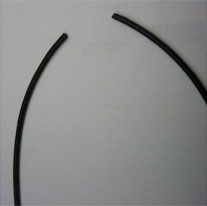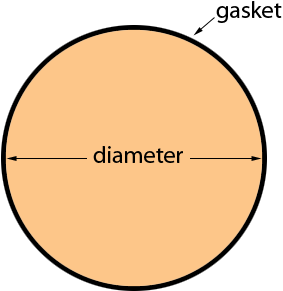SEARCH HOMEMath Central Quandaries & QueriesQuestion from Jorge, a parent: I have an application where I am using a circular silicone gasket. I have the total length but would like to calculate the diameter with the given length. What formula should be used? We receive these gaskets in a roll and cut them about 21 inches and glue the ends together to make it one piece. I would prefer to purchase these already cut and glued but require a diameter. If I had the overall diameter from a 21 inch length gasket, I can look on-line for a part that comes close to the diameter. Thank you in advance, JorgeJorge,

I included the photo you sent of a piece of the gasket.If I understand correctly you want to form the gasket into a circle and you want to know the diameter of the circle as a function length of the gasket, the circumference of the circle.The expression you want is

circumference = π × diameter

where π = 3.1416. Thus

diameter = circumference/π.

Hence for the example you sent with the circumference = 21 inches

diameter = circumference/π = 21/3.1416 = 6.68 inches.

If you don't like decimal parts of inches take the decimal part. 0.68 and multiply by 16 to convert it to sixteenths. Thus 0.68 × 16 = 10.88 so the diameter is 6 11/16 inches.

I hope this helps,
HarleyMath Central is supported by the University of Regina and The Pacific Institute for the Mathematical Sciences.